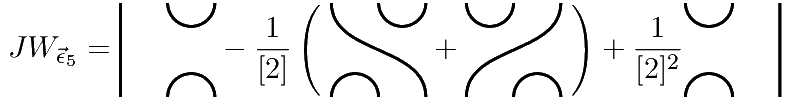## Data

• Title: Cellular structures using $\textbf{U}_q$-tilting modules
• Authors: Henning Haahr Andersen, Catharina Stroppel and Daniel Tubbenhauer
• Status: Pacific J. Math. 292-1 (2018), 21-59. Last update: Mon, 2 Oct 2017 13:46:03 GMT
• ArXiv link: http://arxiv.org/abs/1503.00224
• ArXiv version = 0.99 published version
• LaTex Beamer presentation: Slides1, Slides2, Slides3
• Additional file: File

## Abstract

We use the theory of $\textbf{U}_q$-tilting modules to construct cellular bases for centralizer algebras. Our methods are quite general and work for any quantum group $\textbf{U}_q$ attached to a Cartan matrix and include the non-semisimple cases for $q$ being a root of unity and ground fields of positive characteristic. Our approach also generalize to certain categories containing infinite dimensional modules. As applications, we give a new semisimplicty criterion for centralizer algebras, and recover the cellularity of several known algebras (with partially new cellular bases) which all fit into our general setup.

## A few extra words

One of our main points is that our approach collects cellular structures on a lot of important algebras under one roof (usually the proofs of cellularity of these algebras are spread over the literature). For example, we recover the cellularity of the following interesting algebras.

• The group algebras of the symmetric group $\mathbb{K}[S_d]$ and its corresponding Iwahori-Hecke algebra $\mathcal{H}_d(q)$.
• $\mathfrak{sl}_2$ related algebras like Temperley-Lieb algebras $\mathcal{TL}_d(\delta)$ and others.
• Spider algebras in the sense of Kuperberg.
• The group algebras of the complex reflection groups $\mathbb{K}[\mathbb{Z}/r\mathbb{Z}\wr S_d]$ and its corresponding Ariki-Koike algebra $\mathcal{H}_{d,r}(q)$. In particular, Hecke algebras of type $B$.
• Algebras related to $\mathcal{H}_{d,r}(q)$, e.g. (quantum) rook monoid algebras $\mathcal{R}_{d}(q)$ and blob algebras $\mathcal{BL}_{d}(q,m)$.
• Brauer algebras $\mathcal{B}_{d}(\delta)$ and its quatazation, the BMW algebras $\mathcal{BMW}_{d}(\delta)$.
• Algebras related to $\mathcal{B}_{d}(\delta)$, e.g. walled Brauer algebras $\mathcal{B}_{r,s}(\delta)$.
• More...
Even better: our methods also generalize to categories containing infinite dimensional modules. In particular, to the BGG category $\boldsymbol{\mathcal{O}}$, its parabolic subcategories $\boldsymbol{\mathcal{O}}^{\mathfrak{p}}$ and its quantum cousin $\boldsymbol{\mathcal{O}}_q$. Using this, we recover for example the following cellular structures as well.
• Generalized Khovanov arc algebras.
• $\mathfrak{sl}_n$-web algebras.
• Cyclotomic Khovanov-Lauda Rouquier algebras of type $A$.
• $\begin{xy}(0,0)*{\bigvee_d};(-1,0)*{\bigvee_{\phantom{d}}};\end{xy}$-algebras.
• More...
• Our construction of cellular bases is explicit and can be illustrated in a “bow-tie” diagram. Moreover, for the Temperley-Lieb we obtain the so-called “generalized Jones-Wenzl projectors” as basis elements, e.g. (up to a scalar) such a projector looks like: